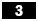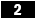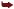Using C Operators

Using C# Operators

C# provides several operators for arithmetic, logical, increment, decrement, and other operations. C# also provides for shorthand notations for common situations such as adding an amount to a variable. In addition, most of the C# operators can be overloaded by the developer. Overloading enables the developer to specify the behavior for the operator when applied to a specific type. Overloading will be covered later in this chapter. Table 3.1 provides a listing of all operators for C#.

Table 3.1. C# Operators

Category

Operator

Description

Arithmetic

+

Yes

-

Subtraction

Yes

*

Multiplication

Yes

/

Division

Yes

%

Modulus remainder

Yes

Logical

&

Bitwise AND

Yes

|

Bitwise OR

Yes

^

Bitwise exclusive OR

Yes

!

Logical negation

Yes

~

Bitwise complement

Yes

&&

Logical AND

Not directly

||

Logical OR

Not directly

Increment/decrement

++

Pre/post increment

Yes

--

Pre/post decrement

Yes

Shift

<<

Binary left shift

Yes

>>

Binary right shift

Yes

Relational

==

Equality

Yes

!=

Not equal

Yes

<

Less than

Yes

>

Greater than

Yes

<=

Less than or equal

Yes

>=

Greater than or equal

Yes

Assignment

=

Assign

No

+=

Not directly

-=

Subtract assign

Not directly

*=

Multiply assign

Not directly

/=

Divide assign

Not directly

%=

Modulus assign

Not directly

&=

Bitwise AND assign

Not directly

|=

Bitwise OR assign

Not directly

<<=

Left-shift assign

Not directly

>>=

Right-shift assign

Not directly

Member access

.

Member access

No

Indexing

[]

Indexing

No, but indexers can be defined

Cast

()

Casting

No, but cast operators can be defined

Conditional

?:

Ternary if statement

No

Object creation

new

Creates an instance of an object

No

Type information

as

Safe cast to specified type

No

is

Used to determine whether a type is of a specified type

No

Overflow

checked

Controls overflow checking context

No

unchecked

Controls overflow checking context

No

*

Pointer dereference

No

->

Pointer member access

No

[]

Pointer indexing

No

&

No

It's important to note the operators for which overloading cannot be performed directly. This category of operators is really shorthand notations for an extended syntax. For example, the expression x += y gets expanded and evaluated as x = x + y; therefore, if the addition operator + is overloaded, that operator will be used in the evaluation of the += operator. Operator overloading is a powerful feature of C# that offers the flexibility to allow for user-defined types to be manipulated as if they were built-in C# types. To explore the power of C# operators, create a new C# console application and name it Operators. Then copy the code from Listing 3.2 into the project. Build and run the project without debugging (Ctrl+F5) to see the output.

////////////////////////////////////////////////////    ///This project demonstrates operator overloading    ///in C#    ////////////////////////////////////////////////////    using System;    namespace Operators {        /// <summary>        /// Mile class will define operators for increment/decrement        /// </summary>        public class Mile {            private int        m_wholePart    = 0;            private int        m_tenthPart    = 0;            public int WholePart { get { return m_wholePart; } set { m_wholePart = value; } }            public int Tenths    { get { return m_tenthPart; } set { m_tenthPart = value; } }            public Mile( ) { }            //operators/// <summary>            /// Increment the tenths of a mile            /// </summary>            static public Mile operator++( Mile mile ) {                mile.m_tenthPart++;                if( mile.m_tenthPart >= 10 ) {                     mile.m_wholePart++;                     mile.m_tenthPart -= 10;                 }                 return mile;            }            /// <summary>            /// Decrement the number of tenths in the mile            /// </summary>            static public Mile operator--( Mile mile ) {                mile.m_tenthPart--;                if( mile.m_tenthPart < 0 ) {                    mile.m_wholePart--;                    mile.m_tenthPart = 10 + mile.m_tenthPart;                }                return mile;            }            /// <summary>            /// Add two mile objects together            /// </summary>            static public Mile operator+( Mile a, Mile b ) {                int tenthPart    = a.m_tenthPart + b.m_tenthPart;                int wholePart = a.m_wholePart + b.m_wholePart;                if( tenthPart >= 10 ) {                    tenthPart = tenthPart - 10;                    wholePart++;                }                Mile result = new Mile( );                result.m_tenthPart    = tenthPart;                result.m_wholePart    = wholePart;                return result;            }            /// <summary>            /// Subtract two different Mile objects            /// </summary>            static public Mile operator-( Mile a, Mile b ) {                Mile result = new Mile( );                result.m_tenthPart    = a.m_tenthPart - b.m_tenthPart;              if( result.Tenths < 0 ) {                   result.m_tenthPart                = 10 - result.m_tenthPart;                   result.m_wholePart                = a.m_wholePart - b.m_wholePart - 1;                 } else {                     result.m_wholePart                = a.m_wholePart - b.m_wholePart;                 }                 return result;           }            /// <summary>            /// Returns the number of feet in a mile when casted to an float value            /// </summary>            static public explicit operator float( Mile mile ) {                float feet = 5280 * mile.m_wholePart;                feet *= ( (float)mile.m_tenthPart / 10.0f );                return feet;            }            /// <summary>            /// override the ToString method and return WholePart.TenthPart            /// </summary>            public override string ToString() {                return string.Format( "{0}.{1}", m_wholePart, m_tenthPart );            }        }        /// <summary>        /// Test harness for operators        /// </summary>        class OperatorTest {            /// <summary>            /// Defines the entry point for the application            /// </summary>            static void Main( ) {                Mile mile = new Mile( );                mile.WholePart    = 5;           //5 miles                mile.Tenths       = 1;           //5.1 miles                mile++;                         //5.2 milesConsole.WriteLine( mile );      //the ToString //method will be invoked onthe Mile class                mile++;                         //5.3 miles                Console.WriteLine( mile );                float feet    = (float)mile;    //get the //number of feet in 5.3 miles                //Display the number of feet in 5.3 miles                Console.WriteLine( "Number of feet in {0} miles is {1}", mile, feet );                Mile mile2    = new Mile( );                mile2.WholePart    = 2;                Mile result = mile - mile2;        //result should equal 3.3 miles                Console.WriteLine( "{0} - {1} = {2}", mile, mile2, result );            }        }    }

Listing 3.2 begins by defining a class called Mile. The Mile class keeps track of whole miles and tenths of a mile. By overloading various operators such as increment, decrement, and addition, and a casting operator, the Mile class acts as if it were a built-in C# type. Walking through the code with the debugger will help to gain an insight as to what is happening during the execution of the code. Place a breakpoint on the shaded lineat line 114 of Listing 3.2. This is the first place where one of the overloaded operators will be invoked. It's important to realize that operators are just syntactic sugar for method calls. The literal translation of line 114 is mile = Mile.operator++( mile ). For now, ignore everything in the listing expect for the operator-overloading code. The rest, such as the properties WholePart and Tenths, and the fields m_wholePart and m_tenths, will be covered in Chapter 6, "Objects and Classes." Right now, just focus on the operators. From line 114, the code executes the overloaded increment operator found on line 29. From there, the m_tenths value is incremented with the same ++ operator that is defined for integer types.Visual C#. NET 2003 Unleashed
ISBN: 672326760
EAN: N/A
Year: 2003
Pages: 316